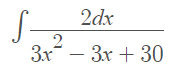# Completing the Square Method (Integrals)

The Completing the square method is one way to solve a quadratic equation. It requires a few more steps than some of the other methods (like the quadratic formula), but it forms a foundation for an integration method of the same name.

Contents:

## Completing the Square Method: Solving Quadratic Equations

Example question: Solve 2x2 + 12x – 18 = 0 by completing the square.

Step 1: Separate the constant term from the other terms by moving it to the right side of the equation. Adding 18 to both sides gives:
2x2 + 12x = 18.

Step 2: Remove any coefficient from x2. For this example, we can get rid of the “2” by dividing:This gives x2 + 6x = 9.

Step 3: Halve the x-coefficient, square it, then add the result to both sides. The x-coefficient is 6, so:

1. Halving the x-coefficient: ½ * 6 = 3
2. Squaring: 32 = 9
3. Adding to both sides: x2 + 6x + 9 = 18.

Step 4: Factor the left side of the equation from Step 3:
Factoring x2 + 6x + 9:

• (x + 3(x + 3) = 18
• (x + 3)2 = 18

I skipped a few factoring steps here, but if you’re a bit rusty, you can view the intermediate steps on Symbolab.

Step 5: Solve for x:

1. Take the square root of both sides:
• √[(x + 3)2] = ±√(18)
• x + 3 = ±√18
2. Subtract 3 from both sides: x = ±√18 – 3

The solution is x = ±√18 – 3, which simplifies to x = -3±3√2.

## Integration of Fractions

The Completing the Square Method is a method of last resort to deal with expressions that can’t be integrated using the “usual” rules like the power rule. Specifically, you use this method when you have a quadratic polynomial in the denominator and no variables in the numerator.

Example question: Find the integral by completing the square:Step 1: Bring the constant in front and rewrite with dx at the end:Step 2: Complete the square for the denominator only (following the above procedure for quadratic equations):That’s it! Now the function is in a form where you can use the usual rules of integration. For this particular function, you can solve it with u-substitution:

1.Substitute u = x – 1
2.Integral Substitution u = 3√3 / √3 v
3.Take out the constant
4.Use the common integral ∫ 1/(v2 + 1) dv = arctan (v)
5.Substitute the original function back in and simplify

CITE THIS AS:
Stephanie Glen. "Completing the Square Method (Integrals)" From StatisticsHowTo.com: Elementary Statistics for the rest of us! https://www.statisticshowto.com/completing-the-square-method-integrals/